Sum( and Seq( commands on your calculator

Can you find the sum of the following series?$\displaystyle \sum_{i=1}^{15}\dfrac{2n+1}{3n-2}$

This is neither an arithmetic nor a geometric series, so you don’t have a formula for it. This would be a tedious problem to do by hand. Fortunately, your graphing calculator can do these problems quickly and efficiently.

There are two functions you need to use on your calculator. The seq( command creates a sequence of terms based on a rule that you give. The sum( command adds together the terms in a sequence. Both functions are found on the LIST menu on your calculator. The seq( command is on the OPS submenu and the sum( command is on the MATH submenu.

To sum a series, you combine the two commands. If you have the new operating system on your calculator, it will prompt you for the entries when you select the seq( command. If you have the old operating system, you need to know the syntax for the command. The syntax for the series above is:

sum(seq((2x + 1)/(3x – 2),x,1,15))

Note that the seq( command has four parameters in the parentheses. From left to right, these are 1) the rule for the nth term of the sequence; 2) the variable name; 3) the first value of the variable; and 4) the final value of the variable. Now all you need to do is type this in to your calculator and let it do the crunching:

The “parent” functions

Do you know your parent functions? I’m surprised every year when some of my students don’t know how to graph some of the elementary functions they’ve learned in class. Your chances for success in precalc and calculus are significantly better if you memorize the shapes of these ten functions. No excuses, no shortcuts. Just learn them.

y = xn (Power functions)

y = |x| (absolute value function)

y = ⌊x⌋ (Greatest integer function)

y = √x

y = 1/x

y= bx (exponential function – growth and decay)

y = log x

y = sin x and y = cos x

y = tan x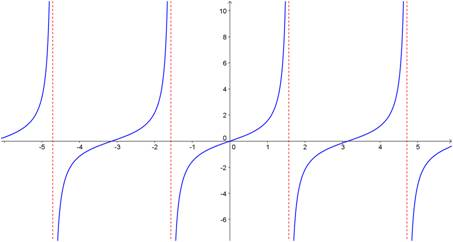Simplifying “3-stack” and “4-stack” fractions

I had a physics student a number of years ago who worked a complicated problem and ended up with the following (I’ve changed the actual numbers to make our work here easier to follow):$\dfrac{(2)(3)}{4}=\dfrac{(5)(6)(7)B}{(8)(9)}$

Of course, his next step was to solve for B. It should be clear to you, as it was to him, that he needed to multiply both sides by 8 and 9 and divide by 5, 6 and 7. That is what he did. But this is how he wrote the solution:$B= \dfrac{9 \cdot \left( \dfrac{ \left(\dfrac{ 8 \cdot \left( \dfrac{(2)(3)}{4} \right)}{5} \right)}{6} \right)}{7}$

His expression was totally correct, and he found the correct value of B, but he made the problem so much harder than he needed to. He created a fraction with five different stacks in it and he needed all those parentheses to keep track of which number was a numerator and which was a denominator.

This is an extreme case of what I see so many students do: they create “3-stack” and “4-stack” fractions all the time when they are simplifying problems. Let’s look at two examples. I’ll solve them with “bad” solutions and then with much smarter solutions (I don’t simplify the answers here because I want to focus on the first step of the solution):

Example 1. Solve:$3x= \dfrac{2}{5}$$x= \dfrac{\dfrac{2}{5}}{3}$

Smarter solution:$x= \dfrac{2}{5 \cdot 3}$

Example 2. Solve:$\dfrac{4}{7}x=\dfrac{2}{5}$$x= \dfrac{\dfrac{2}{5}}{\dfrac{4}{7}}$

Smarter solution:$x= \dfrac{2 \cdot 7}{5 \cdot 4}$

You can see that the smarter solutions are simpler to read and easier to simplify.

It is easy to train yourself to write answers as “2-stack” fractions if you remember one simple rule:

Respect the vinculum.

Um, respect the what?!?

The vinculum. When you write a fraction, the horizontal line that separates the numerator from the denominator is called the vinculum. No one ever talks about it, but it’s a very powerful symbol. It tells you to multiply by every number that is above it and divide by every number that is below it. And it’s as easy as that. So when you are simplifying expressions like the ones in the two examples above or the more complicated example at the beginning of this post, all you need to do is to put numbers that are multiplied above the vinculum and numbers that are divided below the vinculum. And what happens if you are multiplying or diving by a fraction? Then put the numerator on top and the denominator on the bottom if you are multiplying. Flip the fraction over first if you’re dividing (as I did in Example 2). When you do this, you will end up with a 2-stack fraction that can be easily evaluated. Let’s look at the original problem again:$\dfrac{(2)(3)}{4}=\dfrac{(5)(6)(7)B}{(8)(9)}$

To solve for B, you will multiply by 8 and 9, so they go on top. And you will divide by 5, 6 and 7, so they go on the bottom. In one step, you’ve solved for B as follows:$B= \dfrac{2 \cdot 3 \cdot 8 \cdot 9}{5 \cdot 6 \cdot 7}$

Compare that to the monstrosity at the beginning. So much easier!

P.S. You don’t really have to remember the name “vinculum”. Most people don’t know what the line is called and don’t care. Chances are good your math teacher doesn’t even know the term. To make things even more bizarre, when you write a fraction with a slash instead of a horizontal line like this—2/3 – the slash is called a “virgule”.  Most people don’t know that one and don’t care about it either. All you have to remember is that the horizontal line in a fraction tells you to multiply on top and divide on the bottom.

The Most Common Factoring Mistake

I am surprised (and a little disappointed) every year when one of my students tries to simplify a polynomial fraction by cancelling out terms that can’t be cancelled out. For example, when faced with$\dfrac{3x+7}{2x-5}$

inevitably, a student will ask me, “Can I cancel out the x’s like this?”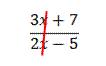Tears well up in my eyes as I explain that no, the x’s do not cancel. I explain patiently why the x’s do not cancel. And very often, the next time I work with that student, he or she will try to cancel out the x’s again. This is the most common factoring mistake I see students make, and it’s not limited to Algebra students. I’ve even seen Calculus student make this error. That usually makes me sob quite loudly.

If you would like to keep me from crying, then you need to learn how to simplify polynomial fractions. It’s quite simple once you understand that terms that are added do not cancel out. Only factors that are multiplied together can cancel. Let’s start by looking at a fraction with numbers and no variables.$\dfrac{210}{462}$

Can this be simplified? Of course. Most students will divide the top and bottom by 2, then by 3, and then by 7, as follows:$\dfrac{210}{462}=\dfrac{105}{231}=\dfrac{35}{77}=\dfrac{5}{11}$

This is correct. But why can you cancel out a 2 and a 3 and a 7? It’s because they are factors of the numerator and the denominator. Let’s do the same problem by completely factoring the top and bottom first:$\dfrac{210}{462}=\dfrac{2\cdot 3 \cdot 5 \cdot 7}{2 \cdot 3 \cdot 7 \cdot 11}=\dfrac{5}{11}$

When you write it out like this, you can see that the 2’s cancel, the 3’s cancel, and the 7’s cancel. And they cancel only because they are factors.

Now let’s try to simplify some polynomial fractions. Start by factoring the numerator and denominator completely, then any like factors will cancel:$\dfrac{2x-4}{6x+14}=\dfrac{2(x-2)}{2(x+7)}=\dfrac{x-2}{x+7}$$\dfrac{4x+8}{12x+24}=\dfrac{4(x+2)}{12(x+2)}=\dfrac{4}{12}=\dfrac{1}{3}$$\dfrac{x^2-1}{x^2+4x+3}=\dfrac{(x+1)(x-1)}{(x+1)(x+3)}=\dfrac{x-1}{x+3}$

Related Rates: Substituting for extra variables

The most complicated type of related rate problems for many students is when there is a variable and you have no information about its rate of change. In these problems, you will know how the variable is related to another variable with a known rate. You can then substitute the variable with the known rate in for the variable with the unknown rate. It sounds difficult, but with a little practice, you can master it.

Example: A water tower in the shape of an inverted cone is being filled at a rate of 2 cubic feet per minute. The tower is 10 feet tall and the radius of the base is 5 feet. How fast is the depth of the water changing when the depth is 4 feet?

Solution: First, we draw a picture. This drawing shows a cross section of the water tower, filled to a height of h.

The variables we know and seek are the following:$\dfrac{dV}{dt}=2; \; \dfrac{dh}{dt}= \, ?$

The variables suggest that the equation we need is the volume of a cone:$V= \dfrac{1}{3} \pi r^2h$

If we take the derivative now, we will end up with a dr/dt term but we are not given any information about how fast the radius is changing. We get around this problem by solving for r in terms of h and substituting. How are r and h related? Notice that the triangle of water in the drawing above is similar to the triangle of the whole water tower. This means we can set up the following relationship:$\dfrac{5}{10}= \dfrac{r}{h} \rightarrow r= \dfrac{h}{2}$

No matter how deep the water is at any time, the radius of the water surface will always be half the height of the water column. Substituting into our equation and simplifying:$V= \dfrac{1}{3} \pi r^2h \rightarrow V= \dfrac{1}{3} \pi \left ( \dfrac{h}{2} \right )^2h \rightarrow V= \dfrac{1}{12} \pi h^3$

Now that r has been eliminated, we can differentiate:$\dfrac{dV}{dt}=\dfrac{\pi}{4}h^2 \dfrac{dh}{dt}$

Plugging in values gives:$2= \dfrac{\pi}{4}(4)^2 \dfrac{dh}{dt}, \qquad \text{so } \dfrac{dh}{dt}= \dfrac{1}{2 \pi} \, ft/min$

(Look for other tips on solving related rate problems on this website if you need help with other aspects of related rate problems.)

Related Rates: Finding missing variables

Often, a related rate problem will require a value for a variable, but you don’t have the value. When this occurs, use the original equation (before you took the derivative) to find the unknown value.

Example: Two cars leave an intersection at the same time. Car A travels north at 60 feet per second. Car B travels east at 80 feet per second. How fast is the distance between them changing 1.0 minute after the cars leave the intersection?

Solution: First draw a picture.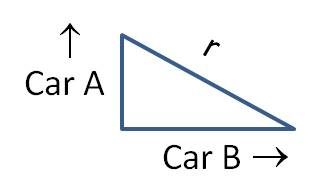It makes sense to let y be the distance car A has traveled from the intersection, and let x be the distance car B has traveled from the intersection. There are two rates given in the problem, and we need to find a third rate:$\dfrac{dy}{dt}=60; \; \dfrac{dx}{dt}=80; \; \dfrac{dr}{dt}= \, ?$

It should be obvious from the picture that the Pythagorean Theorem provides the equation we need:$x^2+y^2=r^2$

Differentiating gives:$2x \dfrac{dx}{dt}+2y \dfrac{dy}{dt}=2r \dfrac{dr}{dt} \rightarrow x \dfrac{dx}{dt}+y \dfrac{dy}{dt}=r \dfrac{dr}{dt}$

To solve for dr/dt, we need to know the value of x, y and r. We can calculate x and y because the cars have been traveling for 60 seconds: x = 4800 ft and y = 3600 ft. To find r, we need to plug these values back into our original equation:$x^2+y^2=r^2 \rightarrow (4800)^2+(3600)^2=r^2 \rightarrow r=6000$

Then plug everything in to solve:$x \dfrac{dx}{dt}+y \dfrac{dy}{dt}=r \dfrac{dr}{dt} \rightarrow (4800)(80)+(3600)(60)=(6000) \dfrac{dr}{dt} \rightarrow \dfrac{dr}{dt}=100 \, ft/s$

(Look for other tips on solving related rate problems on this website if you need help with other aspects of related rate problems.)

Related Rates: Defining Variables

Related rate problems will no longer seem so complicated once you master the art of defining rates as variables. And not just any variables, but variables in the form of derivatives! In other words, express the known and unknown rates as derivatives such as$dV/dt$ or$dA/dt$. Remember that$dA/dt$ means “the rate of change of the Area”. Your task is to find expressions for rates in the problem and “translate” them from English into Calculus. Here are some examples:

• “The radius is increasing at a rate of 3.0 cm/s.”$\dfrac{dr}{dt}=3.0$

•  “If the volume is decreasing at a rate of 1.5 liters per minute…”$\dfrac{dV}{dt}=-1.5$

• “How fast is the ladder falling down the side of the building?”$\dfrac{dy}{dt}= \, ?$

Remember that speed at which something moves is the rate of change of its position. Also, this derivative expresses the unknown we are going to find in the problem.

• “The temperature is dropping by 2.3° per hour.”$\dfrac{dT}{dt}=-2.3$

Note that when a value is falling or decreasing, the value of the derivative is negative.

Once you have defined all your rates as variables, your next step is to write the equation that expresses one variable in terms of the others. However, the equation has to be in terms of the quantities in the numerators, not in terms of the derivatives. Yes, this seems all wrong, but you will get the derivatives when you differentiate each side of the equation. For example, if your two rates are dV/dt and dr/dt, then you need to write an equation that expresses V in terms of r. When you differentiate this equation, you get the related rates equation and can plug in all the values you have.

Example: A hot air balloon in the shape of a sphere is being filled with gas at the rate of 20 cubic meters per minute. How fast is the radius changing when the volume is 288π cubic meters?

Solution: First, find all the rates mentioned in the problem:$\dfrac{dV}{dt}=20; \; \dfrac{dr}{dt}= \, ?$

Now, you need an equation that relates volume (V) to radius (r). Because the balloon is a sphere, use the formula for the volume of a sphere:$V= \dfrac{4}{3} \pi r^3$

Next, differentiate with respect to time:$\dfrac{dV}{dt}=4 \pi r^2 \dfrac{dr}{dt}$

This equation includes r. You need to plug in the value of V in the original equation to solve for r:$V= \dfrac{4}{3} \pi r^3 \rightarrow 288 \pi= \dfrac{4}{3} \pi r^3 \rightarrow 216=r^3 \rightarrow r=6 \, m$

Now plug in all the values to solve for the unknown rate:$\dfrac{dV}{dt}=4 \pi r^2 \dfrac{dr}{dt} \rightarrow 20=4 \pi (6)^2 \dfrac{dr}{dt} \rightarrow \dfrac{dr}{dt}= \dfrac{20}{4 \pi (36)} \approx 0.044 \, m/min$

(Look for other tips on solving related rate problems on this website if you need help with other aspects of related rate problems.)

Related Rates: Getting Started

The most valuable first step when doing a related rate problem is to draw a picture. This helps you figure out the geometry of the problem.

Example: A boat is being towed to a dock by a rope attached to a winch that is five feet higher than the boat. If the winch is pulling the rope at 1.2 m/s, how fast is the boat moving when it is 12 m from the dock?

Solution: It would be very difficult to set up and solve this problem without a quick sketch. But once you draw the picture (and label the components as shown), you can see that a simple right triangle describes the situation.The equation you need is therefore: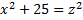Differentiating gives: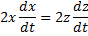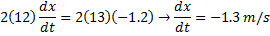So the boat is approaching the dock at 1.3 m/s.

(Look for other tips on solving related rate problems if you need help with some of the details of the solution.)

Simplifying calculus by simplifying equations at each step

Get in the habit of simplifying your equations as you go. If you need to find a second (or higher) derivative, simplify f ′(x) before you take the derivative again. If you are finding the volume of revolution, simplify your integral before you evaluate it. If you are applying l’Hôpital’s rule, simplify your new expression before you plug in the value of x again. If you don’t simplify, you usually make your task much harder.

Example: Find f ″(x).$f(x)= \dfrac{2x+1}{x+1}$

Solution: The first derivative is straightforward:$f'(x)= \dfrac{(x+1)2-(2x+1)1}{(x+1)^2}$

Taking the derivative of this is somewhat messy, unless you simplify first, as follows:$f'(x)= \dfrac{(x+1)2-(2x+1)1}{(x+1)^2}= \dfrac{(2x+2)-(2x+1)}{(x+1)^2}= \dfrac{1}{(x+1)^2}$

Now the second derivative is much easier to evaluate:$f''(x)= \dfrac{-2}{(x+1)^3}$

Optimization problems

Optimization problems can be tamed if you remember one simple idea. The purpose of an optimization problem is to optimize the situation. That is, you want to find where the results are the biggest, or the smallest, or the fastest, or the highest. In other words, if you can find an equation that describes the situation, you are being asked to find the relative minimum or relative maximum of that function. So break these problems down into three steps:

1. Determine what parameter you are optimizing.
2. Write a formula that gives that parameter in terms of an independent variable.
3. Take the derivative to find the relative min and/or max.

Example: What are the dimensions of the rectangle with the largest area that can be inscribed above the x-axis and under the function f(x) = 9 – x2?

Solution: (Step 1) In this case, you are trying to maximize area. So you need (Step 2) a formula that gives the area in terms of the x-variable. Let’s draw a picture: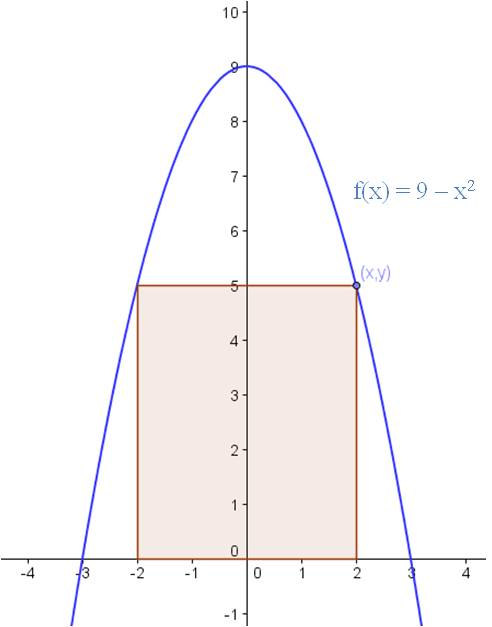We can see from the sketch that if the upper right corner of the rectangle is on the point (x,y), the base of the rectangle is 2x and the height is y. So our first effort at an equation for the area is

A = 2xy.

To finish the problem, we need to express y in terms of x. Since the point is on the parabola, y = 9 – x2. Therefore, the equation we seek is

A = 2x(9 – x2) = 18x – 2x3.

To find the dimensions of the largest possible area, we find the relative maximum of the equation (Step 3).

A’ = 18 – 6x2 = 0 → x2 = 3

Plugging back into the equation for y gives y = 6.

Blue Taste Theme created by Jabox